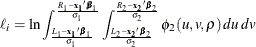The QLIM Procedure

Bivariate Limited Dependent Variable Modeling

The generic form of a bivariate limited dependent variable model is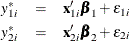where the disturbances,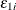and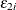, have joint normal distribution with zero mean, standard deviations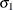and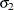, and correlation of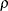.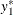and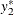are latent variables. The dependent variables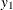and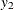are observed if the latent variablesandfall in certain ranges: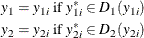is a transformation from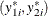to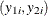. For example, if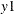and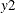are censored variables with lower bound 0, then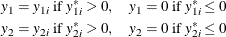There are three cases for the log likelihood of. The first case is that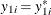and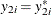. That is, this observation is mapped to one point in the space of latent variables. The log likelihood is computed from a bivariate normal density,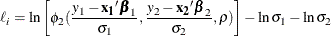where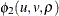is the density function for standardized bivariate normal distribution with correlation,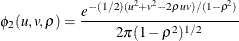The second case is that one observed dependent variable is mapped to a point of its latent variable and the other dependent variable is mapped to a segment in the space of its latent variable. For example, in the bivariate censored model specified, if observed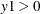and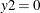, then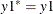and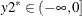. In general, the log likelihood for one observation can be written as follows (the subscript i is dropped for simplicity): If one set is a single point and the other set is a range, without loss of generality, let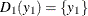and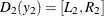,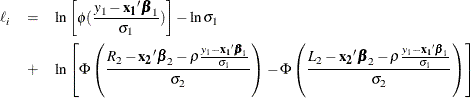whereand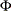are the density function and the cumulative probability function for standardized univariate normal distribution.

The third case is that both dependent variables are mapped to segments in the space of latent variables. For example, in the bivariate censored model specified, if observed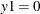and, then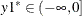and. In general, if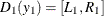and, the log likelihood is